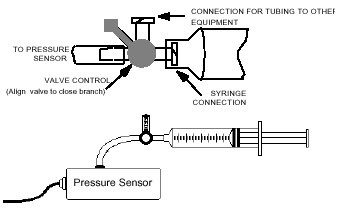# Don’t Push It

The goal of this experiment is to investigate how the gas pressure changes with the volume.

Equipment Needed:

• CBL Unit,
• TI graphing calculator with a unit-to-unit link cable,
• Pressure Sensor with 20 ml gas syringe,Procedure

The picture above shows a pressure sensor attached to something that looks very much like a large hypodermic needle. It consists of a cylinder with a plunger. By pushing the plunger in or pulling it out you can vary the volume of the air in the cylinder. The side of the cylinder is marked with volume in cubic centimeters. The pressure sensor measures the air pressure inside the cylinder.

Before connecting the syringe to the sensor, move the syringe to the 10-mL volume mark. Connect the plastic syringe to the white stem on the end of the Gas Pressure sensor box, with a gentle 1/2 turn. Close the stem that leads into the Pressure Sensor box using the control handle, and set the syringe to any volume setting you like. Then close the open stem (see the figures above). The pressure inside the syringe is now equal to atmospheric pressure at the volume you selected. You are now set to collect pressure-volume data. Take data as you change the volume by moving the syringe. See the CBL guidebook and the sensors’ guide for specific setup instructions on how to hook up the sensor and take readings. The syringe is marked in volume units (cc or mL).

Investigations

• Take and record a series of pressure readings with the plunger set so that the volume in the cylinder is 20, 19, 18, … 5 cubic centimeters.
•  Create a scatter plot (volume vs. gas pressure) for the data using either with your graphing calculator or a spreadsheet.
•  Construct a function that will model the data.
•  From your best-fit equation (function), what is the relationship between volume and gas pressure?
•  Using the function predict the gas pressure at volumes 4 cc, 3 cc, 1 cc?
•  Do you think that it is possible to have an infinitive gas pressure? If yes, how? If not, Why?
•  If P1 is the pressure for the volume V1, and P2 is the pressure for the volume V2, find a relation between P1, V1, P2, and V2.
•  Based on the relation you found above, try to answer the following: Suppose you have a gas with 45.0 ml of volume and have a pressure of 760.mm. If the pressure is increased to 800mm and the temperature remains constant, then according to Boyle’s Law What is the new volume?

Hint: Boyle’s Law states the volume of a definite quantity of dry gas is inversely proportional to the pressure, provided the temperature remains constant.